﻿ 一种启闭绞车的静力学仿真分析

# 一种启闭绞车的静力学仿真分析Statics Simulation Analysis of a Type Hoist Winch

Abstract: A type hoist is made up of a frame, a drum and a drive device. In order to analyze the statics per-formance of the hoist winch, working conditions of the hoist winch are combed and two typical critical working conditions are summed up in this article. Finite Element Method (FEM) is used to analyze the hoist winch strength and stiffness. The results showed that the hoist winch structure strength and static stiffness meet the requirement. The analysis method in this article is of refer-ence value to winch calculation in a certain extent.

1. 引言

2. 工况分析

3. 结构有限元分析

3.1. 材料定义

3.2. 计算模型离散化

3.3. 约束和载荷Table 1. Two typical working conditions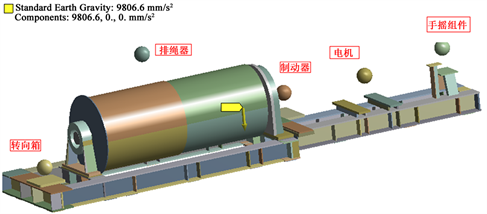Figure 1. Finite Element Model of hoist winch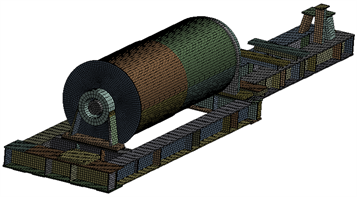Figure 2. FEM Meshing of hoist winch

$\sigma =\frac{2{T}_{\mathrm{max}}\mathrm{exp}\left(-\mu \theta \right)}{Dt} =4.460\mathrm{exp}\left(-\mu \theta \right)$ (1)

$f=2T\cdot \mathrm{exp}\left(-\mu \theta \right)\mu /D=34.878\mathrm{exp}\left(-\mu \theta \right)$ (2)

μ为钢丝绳与卷筒之间摩擦系数，为0.115；为钢丝绳各点的旋转角度加载时选用柱坐标系，坐标轴y向表示θ角。加载如图3所示   。

3.4. 有限元计算

$\left[K\right]\left\{\sigma \right\}=\left[F\right]$ (3)

$\left\{\sigma \right\}$ ——节点位移列阵；

$\left[F\right]$ ——节点载荷列阵。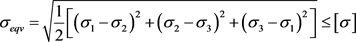(4)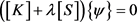(5)

4. 计算结果及分析

4.1. 绞车强度分析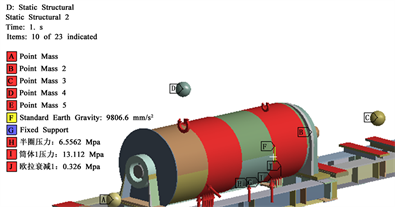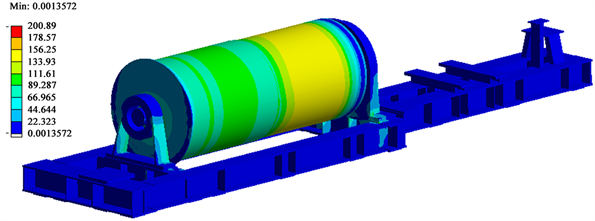Figure 4. Overall stress nephogram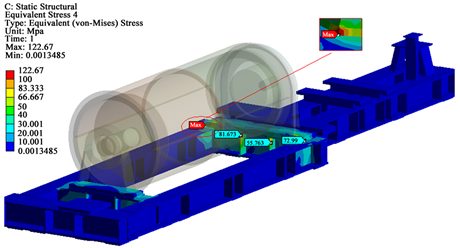Figure 5. Drum stress nephogram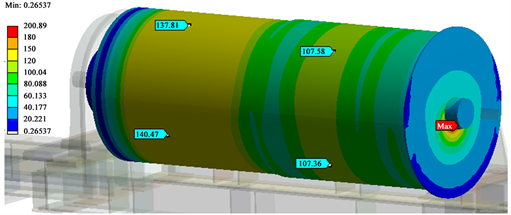Figure 6. Frame stress nephogram

2种工况下绞车的强度和刚度均满足要求。计算结果汇总如表2所示。

4.2. 绞车变形分析Table 2. Finite Element analysis resultsFigure 7. Drum deformation nephogram under working conditions 1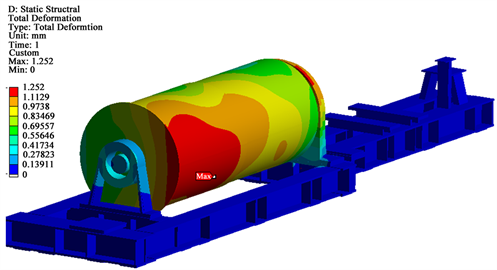Figure 8. Drum deformation nephogram under working conditions 2

5. 结论

 GB/T1955-2008建筑卷扬机[M]. 北京: 中国国家标准化管理委员会, 2008.

 成大先. 机械设计手册[M]. 北京: 化学工业出版社, 2008.

 刘铁钢. 传动卷筒筒体结构有限元分析[D]: [硕士学位论文]. 大连: 大连理工大学, 2014.

 赵义. 基于ANSYS的带式输送机传动卷筒的有限元分析及优化设计[D]: [硕士学位论文]. 邯郸: 河北工程大学, 2013.

 刘鸿文. 材料力学[M]. 第4版. 北京: 高等教育出版社, 2004.

 Gere, J.M. and Timoshenko, S.P. (1984) Mechanics of Materials. Second SI Edition, Van Nostrand Reinhold, New York.

 王新荣, 蒋永波. 有限元法基础及Ansys应用[M]. 北京: 科学出版社, 2008.

 浦广益. ANSYS Workbench基础教程与实例详解[M]. 第二版. 北京: 中国水利水电出版社, 2010.

 王勖成, 邵敏. 有限元基本原理和数值方法[M]. 第二版. 北京: 清华大学出版社, 2003.

Top